# 【Unity3D开发小游戏】超级马里奥

@[TOC]

## 一、前言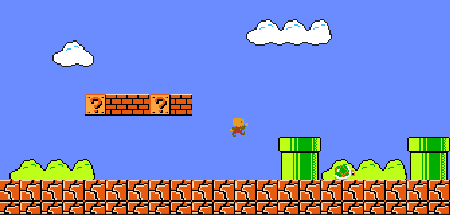## 二、源码

UI资源和源代码请搜索QQ群：1040082875下载

## 三、正文

==Unity5.0.0f4==

### 9.婴儿马里奥

• 闲散

Unity将我们的婴儿马里奥图像分割成几个16x16像素的图像，我们现在可以在项目区看到它们：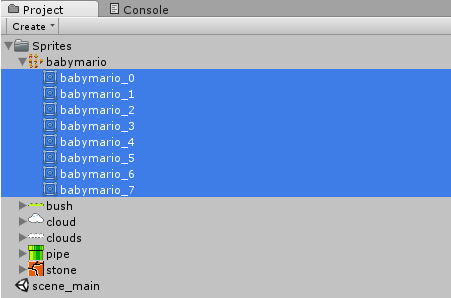### 10.婴儿马里奥动画

• 闲散(第1及2段)
• 跑(第3、4及5段)
• 跳(第6及7段)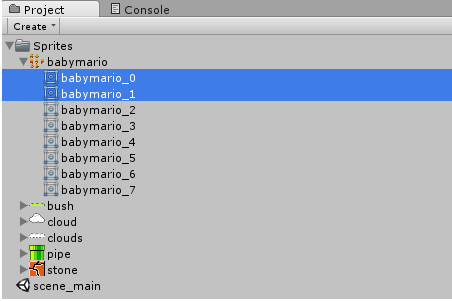然后将它们拖到场景: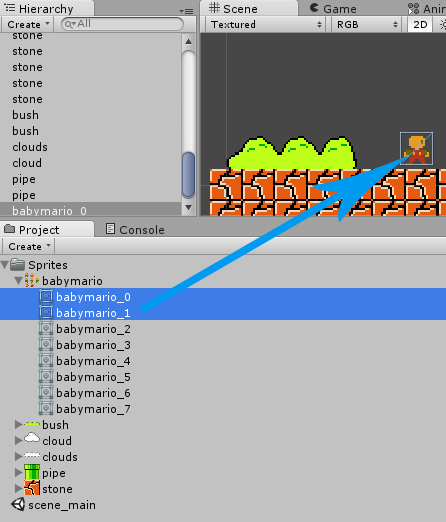将动画保存到BabyMarioAnimation文件夹，命名为Idle.anim：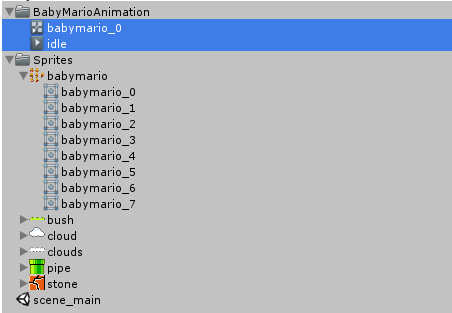第一个文件是动画状态机，它指定诸如动画速度和状态树之类的内容。第二个是动画本身。

### 11.婴儿马里奥动画状态机

• Idel
• Run
• Jump

``````GetComponent<Animator>().SetFloat("Speed", 0);
GetComponent<Animator>().SetBool("Jumping", false);
``````

• Idle到Jump有条件Jumping等于真
• Run到Jump有条件Jumping等于真
• Jump到Idle有条件Jumping等于假

### 13.婴儿马里奥运动脚本

``````using UnityEngine;
using System.Collections;

public class PlayerMovement : MonoBehaviour {

// Use this for initialization
void Start () {

}

// Update is called once per frame
void Update () {

}
}
``````

``````using UnityEngine;
using System.Collections;

public class PlayerMovement : MonoBehaviour {
// Movement Properties
public float moveSpeed = 15;
[Range(0,1)] public float sliding = 0.9f;

// Use this for initialization
void Start () {

}

// Update is called once per frame
void Update () {

}
}
``````

``````using UnityEngine;
using System.Collections;

public class PlayerMovement : MonoBehaviour {
// Movement Properties
public float moveSpeed = 15;
[Range(0,1)] public float sliding = 0.9f;

void FixedUpdate () {

}
}
``````

``````void FixedUpdate () {
// Horizontal Movement
float h = Input.GetAxis("Horizontal");
}
``````

``````Vector2 v = GetComponent<Rigidbody2D>().velocity;
if (h != 0) {
// Move Left/Right
GetComponent<Rigidbody2D>().velocity = new Vector2(h * moveSpeed, v.y);
} else {
// Get slower (Super Mario style sliding motion)
GetComponent<Rigidbody2D>().velocity = new Vector2(v.x * sliding, v.y);
}
``````

``````// Horizontal Movement
float h = Input.GetAxis("Horizontal");
Vector2 v = GetComponent<Rigidbody2D>().velocity;
if (h != 0) {
// Move Left/Right
GetComponent<Rigidbody2D>().velocity = new Vector2(h * moveSpeed, v.y);
} else {
// Get slower (Super Mario style sliding motion)
GetComponent<Rigidbody2D>().velocity = new Vector2(v.x * sliding, v.y);
}
GetComponent<Animator>().SetFloat("Speed", h);
``````

``````// Horizontal Movement
float h = Input.GetAxis("Horizontal");
Vector2 v = GetComponent<Rigidbody2D>().velocity;
if (h != 0) {
// Move Left/Right
GetComponent<Rigidbody2D>().velocity = new Vector2(h * moveSpeed, v.y);
transform.localScale = new Vector2(Mathf.Sign(h), transform.localScale.y);
} else {
// Get slower (Super Mario style sliding motion)
GetComponent<Rigidbody2D>().velocity = new Vector2(v.x * sliding, v.y);
}
GetComponent<Animator>().SetFloat("Speed", Mathf.Abs(h));
``````

``````bool IsGrounded() {
// noobtuts.com isGrounded function

// Get Bounds and Cast Range (10% of height)
Bounds bounds = GetComponent<Collider2D>().bounds;
float range = bounds.size.y * 0.1f;

// Calculate a position slightly below the collider
Vector2 v = new Vector2(bounds.center.x,bounds.min.y - range);

// Linecast upwards
RaycastHit2D hit = Physics2D.Linecast(v, bounds.center);

// Was there something in-between, or did we hit ourself?
return (hit.collider.gameObject != gameObject);
}
``````

``````public float jumpForce = 1200;
``````

``````// Vertical Movement (Jumping)
bool grounded = IsGrounded();
if(Input.GetKey(KeyCode.UpArrow) && grounded)
``````

``````// Vertical Movement (Jumping)
bool grounded = IsGrounded();
if(Input.GetKey(KeyCode.UpArrow) && grounded)
GetComponent<Animator>().SetBool("Jumping", !grounded);
``````

``````using UnityEngine;
using System.Collections;

public class PlayerMovement : MonoBehaviour {
// Movement Properties
public float moveSpeed = 15;
[Range(0,1)] public float sliding = 0.9f;
public float jumpForce = 1200;

bool IsGrounded() {
// noobtuts.com isGrounded function

// Get Bounds and Cast Range (10% of height)
Bounds bounds = GetComponent<Collider2D>().bounds;
float range = bounds.size.y * 0.1f;

// Calculate a position slightly below the collider
Vector2 v = new Vector2(bounds.center.x,
bounds.min.y - range);

// Linecast upwards
RaycastHit2D hit = Physics2D.Linecast(v, bounds.center);

// Was there something in-between, or did we hit ourself?
return (hit.collider.gameObject != gameObject);
}

void FixedUpdate () {
// Horizontal Movement
float h = Input.GetAxis("Horizontal");
Vector2 v = GetComponent<Rigidbody2D>().velocity;
if (h != 0) {
// Move Left/Right
GetComponent<Rigidbody2D>().velocity = new Vector2(h * moveSpeed, v.y);
transform.localScale = new Vector2(Mathf.Sign(h), transform.localScale.y);
} else {
// Get slower (Super Mario style sliding motion)
GetComponent<Rigidbody2D>().velocity = new Vector2(v.x * sliding, v.y);
}
GetComponent<Animator>().SetFloat("Speed", Mathf.Abs(h));

// Vertical Movement (Jumping)
bool grounded = IsGrounded();
if(Input.GetKey(KeyCode.UpArrow) && grounded)
GetComponent<Animator>().SetBool("Jumping", !grounded);
}
}
``````

### 16.道具盒的运动

``````using UnityEngine;
using System.Collections;

public class Box : MonoBehaviour {

// The Curve
public AnimationCurve curve;
}
``````

``````using UnityEngine;
using System.Collections;

public class Box : MonoBehaviour {

// The Curve
public AnimationCurve curve;

void OnCollisionEnter2D(Collision2D coll) {
// collision below?
if (coll.contacts.point.y < transform.position.y) {
// Do stuff...
}
}
}
``````

``````IEnumerator sample() {
// go through curve time
for (float t=0; t < curve.keys[curve.length-1].time; t+=Time.deltaTime) {
// do stuff...

// come back next Update
yield return null;
}
}

void OnCollisionEnter2D(Collision2D coll) {
// collision below?
if (coll.contacts.point.y < transform.position.y)
StartCoroutine("sample");
}
``````

``````float t = 0;

void Update() {
if (t < curve.keys[curve.length-1].time) {
// do stuff..

t += Time.deltaTime;
}
}
``````

``````IEnumerator sample() {
// start position
Vector2 pos = transform.position;

// go through curve time
for (float t=0; t<curve.keys[curve.length-1].time; t+=Time.deltaTime) {
// move a bit
transform.position = new Vector2(pos.x, pos.y + curve.Evaluate(t));

// come back next Update
yield return null;
}
}
``````

### 17.产生金币

``````// The Curve
public AnimationCurve curve;

// The object to spawn
public GameObject spawnPrefab;

IEnumerator sample() {
// start position
Vector2 pos = transform.position;

// go through curve time
for (float t=0; t<curve.keys[curve.length-1].time; t+=Time.deltaTime) {
// move a bit
transform.position = new Vector2(pos.x, pos.y + curve.Evaluate(t));

// come back next Update
yield return null;
}

// spawn an object if any
if (spawnPrefab)
Instantiate(spawnPrefab, transform.position + Vector3.up, Quaternion.identity);
}
``````

``````// The Curve
public AnimationCurve curve;

// The object to spawn
public GameObject spawnPrefab;

// The next box
public GameObject nextPrefab;

IEnumerator sample() {
// start position
Vector2 pos = transform.position;

// go through curve time
for (float t=0; t<curve.keys[curve.length-1].time; t+=Time.deltaTime) {
// move a bit
transform.position = new Vector2(pos.x, pos.y + curve.Evaluate(t));

// come back next Update
yield return null;
}

// spawn an object if any
if (spawnPrefab)
Instantiate(spawnPrefab, transform.position + Vector3.up, Quaternion.identity);

// spawn next one if any, destroy box
if (nextPrefab)
Instantiate(nextPrefab, transform.position, Quaternion.identity);
Destroy(gameObject);
}
``````

### 19.金币的物理

``````using UnityEngine;
using System.Collections;

public class Coin : MonoBehaviour {

void OnTriggerEnter2D(Collider2D coll) {
// Destroy the coin
Destroy(gameObject);
}
}
``````

### 25.敌人动画状态机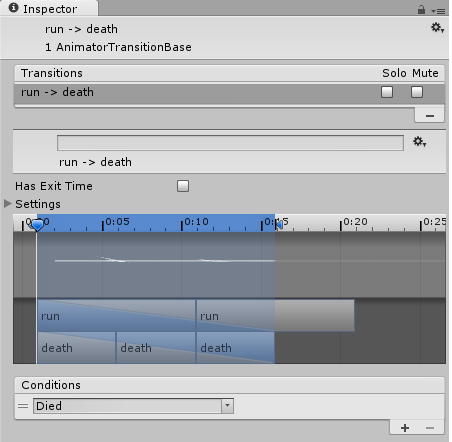注：我们还禁用了Has Exit Time

### 27.敌人的脚本

``````using UnityEngine;
using System.Collections;

public class Enemy : MonoBehaviour {
// Movement Speed
public float speed = 3;

// Current movement Direction
Vector2 dir = Vector2.right;
}
``````

``````void FixedUpdate() {
// Set the Velocity
GetComponent<Rigidbody2D>().velocity = dir * speed;
}
``````

``````void OnTriggerEnter2D(Collider2D coll) {
// Hit a destination? Then move into other direction
transform.localScale = new Vector2(-1*transform.localScale.x,
transform.localScale.y);

// And mirror it
dir = new Vector2(-1 * dir.x, dir.y);
}
``````

• 敌人会死 (如果跳到上面)
• 否则马里奥就会死 (如果水平碰撞)

``````void OnCollisionEnter2D(Collision2D coll) {
// Collided with BabyMario?
if (coll.gameObject.name == "BabyMario") {
// Is the collision above?
if (coll.contacts.point.y > transform.position.y) {
// ToDo kill self
} else {
// ToDo kill BabyMario
}
}
}
``````

``````using UnityEngine;
using System.Collections;

public class Enemy : MonoBehaviour {
// Movement Speed
public float speed = 3;

// Current movement Direction
Vector2 dir = Vector2.right;

// Upwards push force
public float upForce = 800;

void FixedUpdate() {
// Set the Velocity
GetComponent<Rigidbody2D>().velocity = dir * speed;
}

void OnTriggerEnter2D(Collider2D coll) {
// Hit a destination? Then move into other direction
transform.localScale = new Vector2(-1 * transform.localScale.x,
transform.localScale.y);

// And mirror it
dir = new Vector2(-1 * dir.x, dir.y);
}

void OnCollisionEnter2D(Collision2D coll) {
// Collided with BabyMario?
if (coll.gameObject.name == "BabyMario") {
// Is the collision above?
if (coll.contacts.point.y > transform.position.y) {
// Play Animation
GetComponent<Animator>().SetTrigger("Died");

// Disable collider so it falls downwards
GetComponent<Collider2D>().enabled = false;

// Push BabyMario upwards

// Die in a few seconds
Invoke("Die", 5);
} else {
// Kill BabyMario
Destroy(coll.gameObject);
}
}
}

void Die() {
Destroy(gameObject);
}
}
``````

### 28.摘要

• 从动画到物理
• 协同程序
• 动画曲线
• 人物运动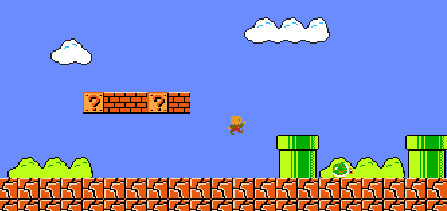和往常一样，现在是读者让游戏尽可能有趣的时候了。

• 增加更多的等级
• 相机跟随
• 更多的敌人
• 一些美妙的声音
• 甚至一个公主